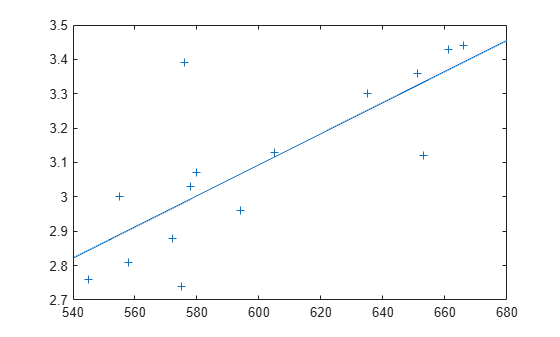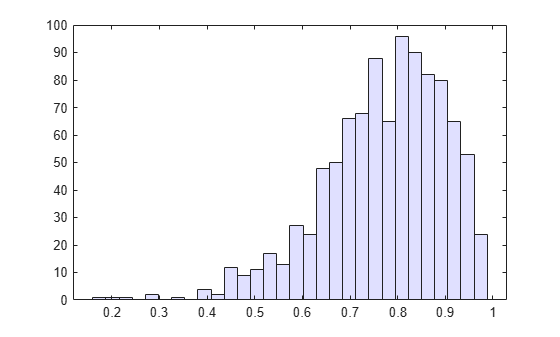## Resampling Statistics

### Bootstrap Resampling

The bootstrap procedure involves choosing random samples with replacement from a data set and analyzing each sample the same way. Sampling with replacement means that each observation is selected separately at random from the original dataset. So a particular data point from the original data set could appear multiple times in a given bootstrap sample. The number of elements in each bootstrap sample equals the number of elements in the original data set. The range of sample estimates you obtain enables you to establish the uncertainty of the quantity you are estimating.

This example from Efron and Tibshirani compares Law School Admission Test (LSAT) scores and subsequent law school grade point average (GPA) for a sample of 15 law schools.

```load lawdata plot(lsat,gpa,'+') lsline```The least-squares fit line indicates that higher LSAT scores go with higher law school GPAs. But how certain is this conclusion? The plot provides some intuition, but nothing quantitative.

You can calculate the correlation coefficient of the variables using the |corr|function.

`rhohat = corr(lsat,gpa)`
```rhohat = 0.7764 ```

Now you have a number describing the positive connection between LSAT and GPA; though it may seem large, you still do not know if it is statistically significant.

Using the `bootstrp` function you can resample the `lsat` and `gpa` vectors as many times as you like and consider the variation in the resulting correlation coefficients.

```rng default % For reproducibility rhos1000 = bootstrp(1000,'corr',lsat,gpa);```

This resamples the `lsat` and `gpa` vectors 1000 times and computes the `corr` function on each sample. You can then plot the result in a histogram.

`histogram(rhos1000,30,'FaceColor',[.8 .8 1])`Nearly all the estimates lie on the interval [0.4 1.0].

It is often desirable to construct a confidence interval for a parameter estimate in statistical inferences. Using the `bootci` function, you can use bootstrapping to obtain a confidence interval for the `lsat` and `gpa` data.

`ci = bootci(5000,@corr,lsat,gpa)`
```ci = 2×1 0.3319 0.9427 ```

Therefore, a 95% confidence interval for the correlation coefficient between LSAT and GPA is [0.33 0.94]. This is strong quantitative evidence that LSAT and subsequent GPA are positively correlated. Moreover, this evidence does not require any strong assumptions about the probability distribution of the correlation coefficient.

Although the `bootci` function computes the Bias Corrected and accelerated (BCa) interval as the default type, it is also able to compute various other types of bootstrap confidence intervals, such as the studentized bootstrap confidence interval.

### Jackknife Resampling

Similar to the bootstrap is the jackknife, which uses resampling to estimate the bias of a sample statistic. Sometimes it is also used to estimate standard error of the sample statistic. The jackknife is implemented by the Statistics and Machine Learning Toolbox™ function `jackknife`.

The jackknife resamples systematically, rather than at random as the bootstrap does. For a sample with `n` points, the jackknife computes sample statistics on `n` separate samples of size `n`-1. Each sample is the original data with a single observation omitted.

In the bootstrap example, you measured the uncertainty in estimating the correlation coefficient. You can use the jackknife to estimate the bias, which is the tendency of the sample correlation to over-estimate or under-estimate the true, unknown correlation. First compute the sample correlation on the data.

```load lawdata rhohat = corr(lsat,gpa)```
```rhohat = 0.7764 ```

Next compute the correlations for jackknife samples, and compute their mean.

```rng default; % For reproducibility jackrho = jackknife(@corr,lsat,gpa); meanrho = mean(jackrho)```
```meanrho = 0.7759 ```

Now compute an estimate of the bias.

```n = length(lsat); biasrho = (n-1) * (meanrho-rhohat)```
```biasrho = -0.0065 ```